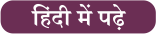Latest SSC jobs   »   Government Jobs 2022   »   Percentage Formula

# Percentage Formula, How to Calculate Percentage?

## Percentage Formula

Percentage: The percentage is used to find out the share or amount of something in terms of 100. In the simplest form, percent means per hundred. Percentages are a portion or fraction of 100 and it is denoted by the symbol ‘%‘, the percentage is majorly used to compare and find out ratios. The percentage is an interesting topic that helps you to calculate interest in your daily life. Various competitive examinations such as SSC, Railway, Banking, CAT, MAT, etc ask the percentage questions in its aptitude section.

## Percentage Calculator

In this article, we are providing the basic definition of a percentage along with the formula of percentage and percentage questions which helps you with a percentage calculator and increase your score in the government competitive exams. You can learn shortcut percentage methods, and formulas to solve the questions on this topic. To score good marks in any exam, it is necessary to have knowledge of the important topics and clear the basic concepts.

Get free notes on Maths

## Percentage Meaning/ Percentage Definition

Percent means “for every 100” or “out of 100.” The (%) symbol is a quick way to write a fraction with a denominator of 100. For example, instead of saying “Ram scored 70 out of 100,” we say “Ram scored 70%”. The percentage can be repressed in decimal form also like 67.9% or 0.72%.## Percentage

The formula to calculate Percentage is very simple.

### Percentage = (Value ⁄ Total Value) × 100

Now let’s take a look at a few examples using this formula.

## How to Calculate Percentage?

Here we are providing some examples to give you the samples of how to calculate percentages using the percentage formula. These questions will help you to understand the Percentage, and percentage formula using a percentage calculator.

### Percentage Questions

##### Example 1: There are 200 students in a class. Out of them, 90 are girls. Find the percentage of girls in the class?

Solution: We are given,

Total students in the class=200

Girls in the class = 90

Percentage of girls in the class = (Girls in the class⁄ Total no. of students) × 100

Percentage of girls in the class = (90/200)x100 = 45%

##### Example 2: The price of a $1.50 candy bar is increased by 25%. Calculate the new price? Solution: We are given, Price of the Candy=$1.50

Increase in the price of candy= 25% or 25/100

New Price of candy= Old Price + increase in price

New Price of candy= 1.50 +25/100

New Price of candy= 1.50 + 1.50 ×.25

New Price of candy= $1.875 ##### Example 3: Radha earns a monthly salary of$1200. She spends $280/month on food. What percent of the monthly salary does she save? Solution: Monthly salary of Radha=$1200

Salary saved by Radha = $(1200 – 280) =$920

The fraction of salary she saves = 920/1200

Percentage of salary she saves = (920/1200)×100 = 920/12 = 76.667 %

Q. What symbol is used to denote ‘percentage’?
Ans: ‘%’ symbol is used to denote the percentage.

Q. What does the word percentage mean?
Ans: Percent means “for every 100” or “out of 100.”

Q. If Sukhbeer sold 12 pens for a rupee and he had a loss of 20%. How many pens he sold for a rupee if he wants to make a profit of 20%.
Ans: If he wants to make a profit of 20%. He must sell 8 pens for a rupee.

Q. What is the formula for Percentage?

Ans: The formula to calculate the percentage of a number out of another number is: Percentage = (Original number/Another number) x 100#### Congratulations!General Awareness & Science Capsule PDFIncorrect details? Fill the form again here

General Awareness & Science Capsule PDF

Thank You, Your details have been submitted we will get back to you.
•Mathematics Quiz For RRB NTPC : 14th Feb...
•Mathematics Quiz For RRB NTPC : 13th Feb...
•Quantitative Aptitude For SSC CHSL : 6th...
•Quantitative Aptitude Quiz SSC CHSL 4th ...
•Quantitative Aptitude Quiz for SSC CHSL ...
•Quantitative Aptitude Quiz for SSC CHSL ...